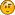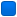###Author Topic: imprecise calculation  (Read 746 times)

####matze

• Member
•• Posts: 4##### imprecise calculation
« on: August 27, 2019, 09:23:41 am »
Hello, I have written a function that does not work correctly, because the function is expected to be inaccurate, which is not due to the source code. It also calculates too imprecisely if I choose the floating point model precise.

I have developed a test program that calls this function:
Code: [Select]
`#include <stdio.h>#include <math.h>static unsigned short int getDecimalPlaces(double value){    unsigned short int result=0;    double currentValue=value>=0.0?value:-value;    currentValue=currentValue-floor(value);    while(currentValue!=0.0)    {        result++;        currentValue*=10;        double floorValue=floor(currentValue);        currentValue-=floorValue;    }    return result;}int main(int argc, char *argv[]){    double value1=99999.999999; //double value with 6 decimal places    unsigned int decimalChars=getDecimalPlaces(value1);    printf("%.6f has %u decimal places\n",value1,decimalChars);    return 0;}`
When running the test program, the following appears in the console:
99999.999999 has 36 decimal placesIs there a way to configure Pelles C so that the function getDecimalPlaces works correctly?
« Last Edit: August 27, 2019, 09:31:35 am by matze »

####TimoVJL

• Global Moderator
• Member
••• Posts: 1882##### Re: imprecise calculation
« Reply #1 on: August 27, 2019, 11:23:08 am »
I don't think it's a Pelles C bug.
Test your example with other compilers and check actual and immediate values.
https://en.wikipedia.org/wiki/Double-precision_floating-point_format

May the source be with you